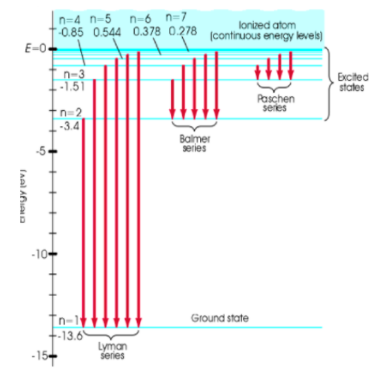# Problem: Determine the frequency of the fourth Lyman line (n = 5 to n = 1transition) using the figure below.  What photon energy does that correspond to?

🤓 Based on our data, we think this question is relevant for Professor Keyes' class at BU.

###### Problem Details

Determine the frequency of the fourth Lyman line (n = 5 to n = 1transition) using the figure below.  What photon energy does that correspond to?